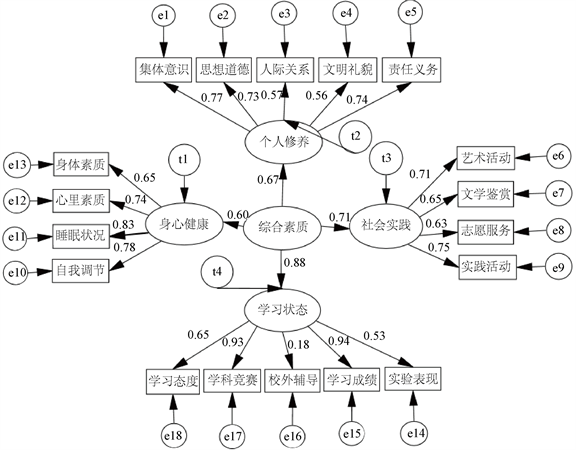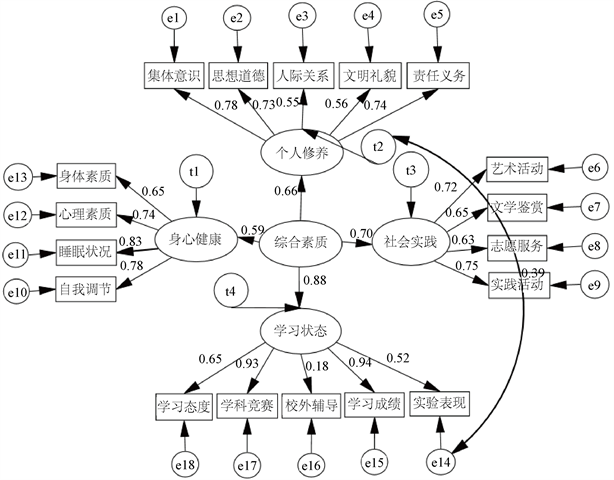﻿ 基于结构方程模型的高中生综合素质评价实证分析

# 基于结构方程模型的高中生综合素质评价实证分析Empirical Analysis on Comprehensive Quality Evaluation of High School Students Based on Structural Equation Model

Abstract: The evaluation index system of comprehensive quality for high school students is established, and the structural equation model of comprehensive evaluation is established through the index system. In order to study this method, the relevant data are obtained from two middle schools in Chongqing by means of questionnaire survey. Based on the survey data and model, this paper makes an empirical study on the comprehensive quality of the students in the two middle schools. The results show that it is effective to quantify the comprehensive quality of senior high school students from four aspects, namely physical and mental health, personal cultivation, social practice and learning state. Among the four aspects, learning state has the largest weight value, and the weight value is 0.31. The examination result is only one aspect of the comprehensive evaluation. High test score does not mean that the comprehensive quality score is also high. Similarly, the high score of comprehensive quality does not mean that the scores of the physical and mental health, personal cultivation, social practice and learning state are high. The charm of comprehensive evaluation lies in the objective evaluation of comprehensive quality based on various factors of high school students.

1. 引言Table 1. A list of comprehensive quality assessment indicators for high school students in some studies

2. 高中生综合素质评价指标体系的构建与修正

2.1. 综合评价指标体系的建立依据

2.2. 评价体系的初步建立

Table 2. Index of evaluation of comprehensive quality of high school students

2.3. 高中生综合素质评价调查问卷

2.4. 综合评价体系的检验与修正Table 3. Cronbach coefficient of initial comprehensive evaluation index systemTable 4. Revised Cronbach coefficient

3. 高中生综合素质评价结构方程模型

3.1. 结构方程模型

$\left\{\begin{array}{c}\eta =B\eta +\Gamma \xi +\zeta \\ x={\Lambda }_{x}\xi +\delta \text{\hspace{0.17em}}\text{\hspace{0.17em}}\text{\hspace{0.17em}}\text{\hspace{0.17em}}\text{ }\text{\hspace{0.17em}}\text{\hspace{0.17em}}\text{\hspace{0.17em}}\\ y={\Lambda }_{y}\eta +\epsilon \text{\hspace{0.17em}}\text{\hspace{0.17em}}\text{\hspace{0.17em}}\text{ }\text{ }\text{ }\end{array}$ (1)

3.2. 模型识别与估计Figure 1. Initial path map of comprehensive quality evaluation for high school students

3.3. 模型拟合与修正Table 5. Model fitting index and test valueFigure 2. Finalpath map of comprehensive quality evaluation for high school students

3.4. 结果分析Table 6. Evaluation index system standardization path coefficient and weight

$\eta =0.21×{\eta }_{1}+0.23×{\eta }_{2}+0.25×{\eta }_{3}+0.31×{\eta }_{4}$ (2)

4. 对重庆两中学的学生学习情况的评价分析Table 7. Scores of the top 20 students

5. 结论

 马世晔. 从国外教育评价制度看我国基础教育评价体系的建立[J]. 中国考试, 2008(5): 13-18.

 孟文娉. 国外高考招生过程中综合素质评价的经验及启示[J]. 教育实践与研究, 2013(5): 9-10.

 周先进, 张睦楚. 高考改革: 高中生综合素质评价的“可为”与“难为”[J]. 全球教育展望, 2014, 43(7): 101-111.

 崔允漷, 柯政. 关于普通高中学生综合素质评价研究[J]. 全球教育展望, 2010, 39(9): 3-8.

 刘坚. 基于多元统计法的综合素质评价模型研究[J]. 曲阜师范大学学报, 2005, 37(3): 19-22.

 邓志勇. 构建学生综合素质评价体系的策略研究[J]. 学校管理与发展, 2010(6): 16-19.

 张东, 张恺, 等. 青少年综合素质的多层次评价[J]. 学术论坛, 2014, 8(8): 182-182.

 郑晓丹, 崔玉平. 京沪浙普通高中生综合素质评价方案的对比分析[J]. 滁州学院学报, 2017, 5(19): 103-107.

 陆炳荣, 杨大忠. 高考综合改革形式下高中生综合素质评价实践探索——以浙江省桐乡市高级中学为例[J]. 中国考试, 2018(2): 30-36.

 王咸伟, 徐晓东, 等. 信息化环境下中小学生综合素质评价指标体系构建[J]. 课程与教学, 2019(1): 67-75.

 李莉. 基于冰山模型探索高校综合评价招生模式[J]. 教育理论研究, 2016(21): 117-118.

Top